In this article, we are going to list the top 10 Machine Learning books that you should read before you start with it. As you know, Machine Learning is a combination of statistic techniques that help computers learn stuff done by humans. This way we are achieving some results with superhuman precision.

Now, as we all know, in order to understand the basics of Machine Learning, it’s nice to have some knowledge in different areas of “Mathematics”.

Before you start reading these top 10 books before starting with Machine Learning, we want to show you two other related articles that you will find very helpful.

We made an article about the essential mathematical skills to get a job as a Data Scientist/Machine Learning Engineer, where we discuss the essential mathematical skills concerning Machine Learning and Data Science.

We also have an article where I talk about my experience with a job interview about Data Science and Machine Learning Engineering.

Since you love reading books, don’t forget to check out these top 15 powerful short Artificial Intelligence books.

# Top 10 books you should read before you dive into Machine Learning

## 1. The Hundred-Page Machine Learning Book by Andriy Burkov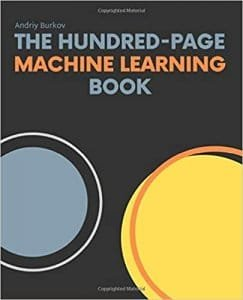This book provides you with everything you need to know about before you dive into Machine Learning. Widely recognized as one of the best books to get you started with the leading branch of the IT industry.

Тhis book will help you learn about: Supervised and unsupervised learning, support vector machines, neural networks, ensemble methods, gradient descent, cluster analysis, and dimensionality reduction, autoencoders and transfer learning, feature engineering, and hyperparameter tuning! Math, intuition, illustrations, all in just a hundred pages!

The author of this book is Andriy Burkov and you can read more information on the official The Hundred-Page Machine Learning Book website.

## 2. Discrete Mathematics and Its Applications 7th Edition by Kenneth Rosen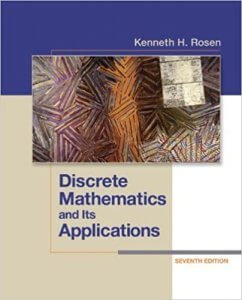Discrete Mathematics and its Applications, Seventh Edition, is intended for one- or two-term introductory discrete mathematics courses taken by students from a wide variety of majors, including computer science, mathematics, and engineering.

This book will complete your Discrete Math journey by showing you an in-depth explanation of different concepts studied in this field, like:

• Sets, subsets, power sets
• Counting functions
• Proof techniques like induction, contradiction
• Basics of inductive, deductive, and propositional logic
• Data structures like arrays, stacks, queues, hash tables, trees
• Graphs and their properties like: connected components, degree, graph coloring, graph traversal, searching methods and algorithms

## 3. Calculus 10th Edition by Howard Anton, Irl C. Bivens, Stephen Davis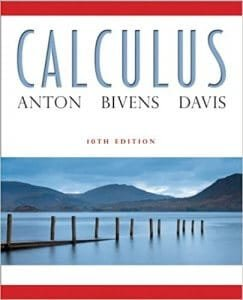Calculus, Tenth Edition excels in increasing student comprehension and conceptual understanding of the mathematics, excellent exercises and examples and appropriate level, while incorporating more skill and drill problems.

## 4. Calculus: An Intuitive and Physical Approach by Morris Kline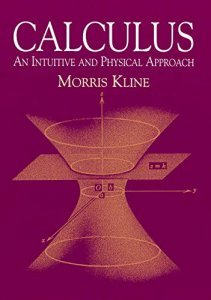This book offers an application-oriented introduction relates to the subject as closely as possible to science. In-depth explorations of the derivative, the differentiation and integration of the powers of x, and theorems on differentiation and antidifferentiation lead to a definition of the chain rule and examinations of trigonometric functions, logarithmic and exponential functions, techniques of integration, polar coordinates, much more.

## 5. Linear Algebra and its Applicationsby David C. Lay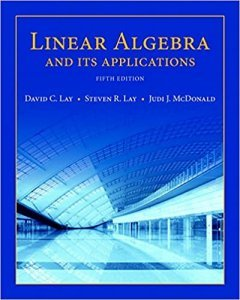This book offers traditional linear algebra texts relatively easy for students during the early stages as material is presented in a familiar, concrete setting. This book will help you easily understand the concepts of Linear Algebra, like:

• Matrices and vectors: scalar multiplication, linear transformation, transpose, calculating the determinant
• Inner and outer products, matrix multiplication rule
• Different matrices types like: square matrix, identity matrix, triangular matrix, an idea about sparse and dense matrix, unit vectors, symmetric matrix
• Matrix factorization concept, Gauss-Jordan elimination, linear system of equation
• Vector space, basis, span, orthogonality, orthonormality, linear least square

## 6. Linear Algebra Done Right  by Sheldon Axler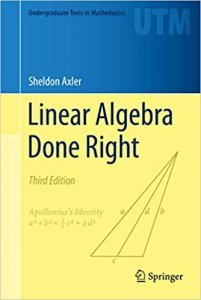The book focuses on the central goal of linear algebra: understanding the structure of linear operators on finite-dimensional vector spaces. It contains a variety of interesting exercises in each chapter helps students understand and manipulate the objects of linear algebra. As the book above, here you will learn the main concepts in Linear Algebra.

## 7. Applied Statistics and Probability for Engineers by D.C. Montgomery, G.C. RungerThis book will explain to you how to use statistical methods to design and develop new products, and new manufacturing systems and processes. You’ll gain a better understanding of how these methods are used in everyday work and get a taste of practical engineering experience through real-world, engineering-based examples, and exercises.

## 8. An Introduction to Statistical Learning: with Applications in R by Gareth James, Daniela Witten, Trevor Hastie, Robert Tibshirani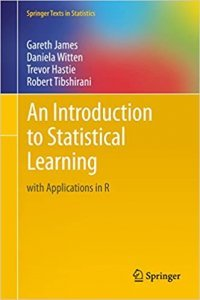An Introduction to Statistical Learning provides an accessible overview of the field of statistical learning, an essential toolset for making sense of the vast and complex data sets. This book presents some of the most important modeling and prediction techniques, along with relevant applications.

Topics include linear regression, classification, tree-based methods, support vector machines, clustering, and more. Color graphics and real-world examples are used to illustrate the methods presented. Since the goal of this textbook is to facilitate the use of these statistical learning techniques by practitioners in science, industry, and other fields, each chapter contains a tutorial on implementing the analyses and methods presented in R, an extremely popular open-source statistical software platform.

## 9. Artificial Intelligence: A Modern Approach by Stuart Russell, Peter Norvig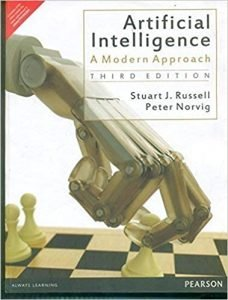This book offers the most comprehensive, up-to-date introduction to the theory and practice of artificial intelligence. Number one in its field, this textbook is ideal for one or two-semester, undergraduate, or graduate-level courses in Artificial Intelligence.

It is the base of every AI course, and it is popular in academic societies across many prestigious universities around the world. We warmly suggest you read this book, as it will get you familiar with many concepts, like Supervised, Unsupervised and Reinforcement Learning, Robotics, Graph search algorithms like (SMA*, A*,  etc).

## 10. Python Crash Course, 2nd Edition: A Hands-On, Project-Based Introduction to Programming by Eric Matthes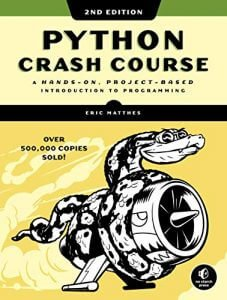Readers will learn how to create a simple video game, use data visualization techniques to make graphs and charts, and build and deploy an interactive web application.

Python Crash Course, 2nd Edition teaches beginners the essentials of Python quickly so that they can build practical programs and develop powerful programming techniques.

We put this book here because Python is the most popular language when it comes to Machine Learning, it is very easy to learn and has tons of useful libraries.

You can use it to implement the algorithms that you will learn in the previous book.

## 11. Python Data Science Handbook: Essential Tools for Working with Data by Jake VanderPlas (Bonus)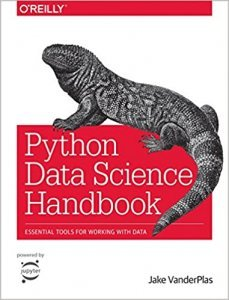For many researchers, Python is a first-class tool mainly because of its libraries for storing, manipulating, and gaining insight from data. Several resources exist for individual pieces of this data science stack, but only with the Python Data Science Handbook do you get them all—IPython, NumPy, Pandas, Matplotlib, Scikit-Learn, and other related tools.

Working scientists and data crunchers familiar with reading and writing Python code will find this comprehensive desk reference ideal for tackling day-to-day issues: manipulating, transforming, and cleaning data; visualizing different types of data; and using data to build statistical or machine learning models. Quite simply, this is the must-have reference for scientific computing in Python.

We will recommend you to go through this book, since it will get you prepared for the more complex tools used in Machine Learning, as well as get you familiar with tools like NumPy, Pandas, Matplotlib, etc, that you are going to use in basically every single model you are going to build.

## Conclusion

So, here are our top 10 essential books that you should read in order to start with Machine Learning. If you feel like there are better choices, go for it, these are the books that we’ve used at university, so we taught it is fair to mention them for you.

If you don’t like to read books, you can find many of the chapters online in video format on YouTube, or on some other fellow sites.

We hope that this stack of books does not intimidate you. We know it looks like a lot, but once you start with those, you are going to love it.

Like with every post we do, we encourage you to continue learning, trying, and creating.